## 2010年10月10日 星期日

### 懶人的 VBA 程式寫作法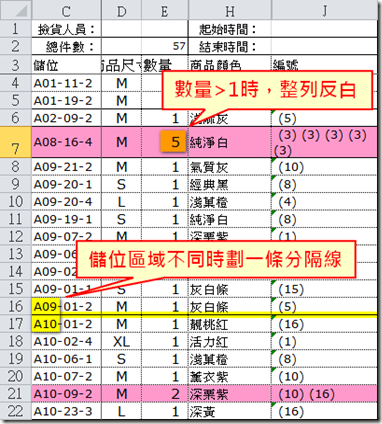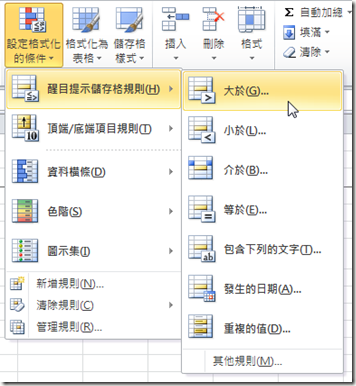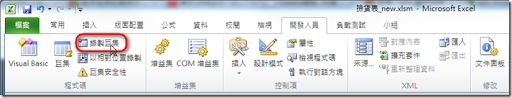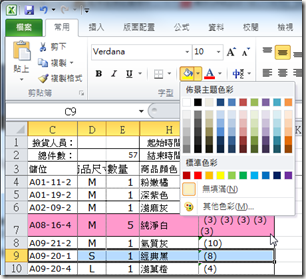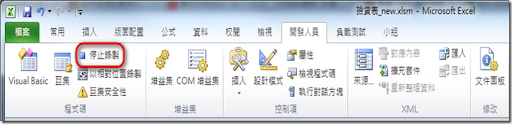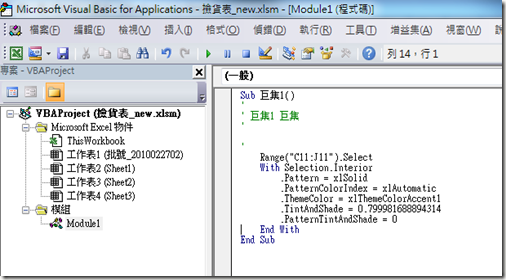```Private Sub Worksheet_Change(ByVal Target As Range)
' 整批貼上時 Range 的左上角是 A4
If Target.row = 4 And Target.Column = 1 Then
FormatAll
End If

' 如果直接改數量...
If Target.row >= 4 And Target.Column = 5 Then
FormatRow Range("\$A" & Target.row & ":\$J" & Target.row)
End If
End Sub

Function FormatAll()
Dim row As Integer
row = 4

Dim continue As Boolean
continue = True

While (continue)
If Cells(row, 1) = "" Then
' 一直處理到沒有批號的那列
continue = False
Else
FormatRow Range("\$A" & row & ":\$J" & row)
row = row + 1
End If
Wend
End Function

Function FormatRow(Target As Range)
' 數量大於1 整列給底色
If Cells(Target.row, 5) > 1 Then
Target.Interior.Color = RGB(255, 153, 204)
Else
Target.Interior.Pattern = xlNone
End If

' 儲位區域不同上方畫實線 (畫雙實線怕列印會換頁)
If Target.row > 4 Then
If Left(Cells(Target.row, 3), 3) <> Left(Cells(Target.row - 1, 3), 3) Then
With Target.Borders(xlEdgeTop)
.LineStyle = xlContinuous
.ColorIndex = 0
.Weight = xlThin
End With
Else
With Target.Borders(xlEdgeTop)
.LineStyle = xlContinuous
.ColorIndex = 0